# Separating hyperplane tutorial¶

This is a very introductory tutorial, showing how a classification task (in this case, deciding whether people are sumo wrestlers or basketball players, based on their height and weight) can be viewed as drawing a decision boundary in a feature space. It shows how to plot the data, calculate the weights of a simple linear classifier, and see how the resulting classifier carves up the feature space into two categories.

Note

This tutorial was originally written by Rajeev Raizada for Matlab and was ported to Python by the PyMVPA authors. The original Matlab code is available from: http://www.dartmouth.edu/~raj/Matlab/fMRI/classification_plane_tutorial.m

Let’s look at a toy example: classifying people as either sumo wrestlers or basketball players, depending on their height and weight. Let’s call the x-axis height and the y-axis weight

sumo_wrestlers_height = [ 4, 2, 2, 3, 4 ]
sumo_wrestlers_weight = [ 8, 6, 2, 5, 7 ]
basketball_players_height = [ 3, 4, 5, 5, 3 ]
basketball_players_weight = [ 2, 5, 3, 7, 3 ]


Let’s plot this.

import pylab as pl
pl.plot(sumo_wrestlers_height, sumo_wrestlers_weight, 'ro',
linewidth=2, label="Sumo wrestlers")
pl.xlim(0, 6)
pl.ylim(0, 10)
pl.xlabel('Height')
pl.ylabel('Weight')
pl.legend()


Let’s stack up the sumo data on top of the basketball players data.

import numpy as np

# transpose to have observations along the first axis
sumo_data = np.vstack((sumo_wrestlers_height,
sumo_wrestlers_weight)).T
# same for the baskball data
# now stack them all together


In order to be able to train a classifier on the input vectors, we need to know what the desired output categories are for each one. Let’s set this to be +1 for sumo wrestlers, and -1 for basketball players.

# creates: [  1,  1,  1,  1,  1, -1, -1, -1, -1, -1]
all_desired_output = np.repeat([1, -1], 5)


We want to find a linear decision boundary, i.e. simply a straight line, such that all the data points on one side of the line get classified as sumo wrestlers, i.e. get mapped onto the desired output of +1, and all the data points on the other side get classified as basketball players, i.e. get mapped onto the desired output of -1.

The equation for a straight line has this form: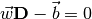were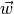is a weight vector,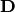is the data matrix, and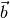is the offset of the dataset form the origin, or the bias. We’re not so interested for now in, so we can get rid of that by subtracting the mean from our data to get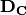the per-column (i.e. variable) demeaned data that is now centered around the origin.

zero_meaned_data = all_data - all_data.mean(axis=0)


Now, having gotten rid of that annoying bias term, we want to find a weight vector which gives us the best solution that we can find to this equation: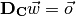were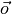is the desired output, or the class labels. But, there is no such perfect set of weights. We can only get a best fit, such that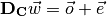where the error term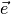is as small as possible.

Note that our equationhas exactly the same form as the equation from the tutorial code in http://www.dartmouth.edu/~raj/Matlab/fMRI/design_matrix_tutorial.m which is: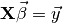where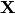was the design matrix,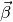the sensitivity vector, and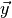the voxel response.

The way we solve the equation is exactly the same, too. If we could find a matrix-inverse of the data matrix, then we could pre-multiply both sides by that inverse, and that would give us the weights: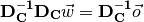The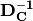andterms on the left would cancel each other out, and we would be left with: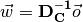However, unfortunately there will in general not exist any matrix-inverse of the data matrix. Only square matrices have inverses, and not even all of them do. Luckily, however, we can use something that plays a similar role, called a pseudo-inverse. In Numpy, this is given by the command pinv. The pseudo-inverse won’t give us a perfect solution to the above equation but it will give us the best approximate solution, which is what we want.we have this equation:

# compute pseudo-inverse as a matrix
pinv = np.linalg.pinv(np.mat(zero_meaned_data))
# column-vector of observations
y = all_desired_output[np.newaxis].T

weights = pinv * y


Let’s have a look at how these weights carve up the input space A useful command for making grids of points which span a particular 2D space is called “meshgrid”

gridspec = np.linspace(-4, 4, 20)
input_space_X, input_space_Y = np.meshgrid(gridspec, gridspec)

# for the rest it is easier to have weights as a simple array, instead
# of a matrix
weights = weights.A

weighted_output_Z = input_space_X * weights + input_space_Y * weights


The weighted output gets turned into the category-decision +1 if it is greater than 0, and -1 if it is less than zero. Let’s plot the decision surface color-coded and then plot the zero-meaned sumo and basketball data on top.

pl.figure()
pl.pcolor(input_space_X, input_space_Y, weighted_output_Z,
cmap=pl.cm.Spectral)
pl.plot(zero_meaned_data[all_desired_output == 1, 0],
zero_meaned_data[all_desired_output == 1, 1],
'ro', linewidth=2, label="Sumo wrestlers")
pl.plot(zero_meaned_data[all_desired_output == -1, 0],
zero_meaned_data[all_desired_output == -1, 1],
pl.xlim(-4, 4)
pl.ylim(-4, 4)
pl.colorbar()
pl.xlabel('Demeaned height')
pl.ylabel('Demeaned weight')
pl.title('Decision output')
pl.legend()

from mvpa2.base import cfg


The full source code of this example is included in the PyMVPA source distribution (doc/examples/hyperplane_demo.py).